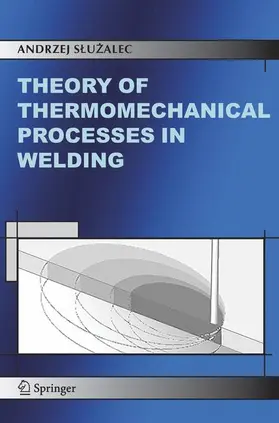# SluzalecTheory of Thermomechanical Processes in Welding

1. Auflage. Softcover version of original hardcover Auflage 2005, 174 Seiten, Kartoniert, Paperback, Format (B × H): 160 mm x 240 mm, Gewicht: 304 g
ISBN: 978-90-481-6762-3
Verlag: Springer Netherlands

## SluzalecTheory of Thermomechanical Processes in Welding

The main purpose of this book is to provide a unified and systematic continuum approach to engineers and applied physicists working on models of deformable welding material. The key concept is to consider the welding material as an thennodynamic system. Significant achievements include thermodynamics, plasticity, fluid flow and numerical methods. Having chosen point of view, this work does not intend to reunite all the information on the welding thermomechanics. The attention is focused on the deformation of welding material and its coupling with thermal effects. Welding is the process where the interrelation of temperature and deformation appears throughout the influence of thermal field on material properties and modification of the extent of plastic zones. Thermal effects can be studied with coupled or uncoupled theories of thermomechanical response. A majority of welding problems can be satisfactorily studied within an uncoupled theory. In such an approach the temperature enters the stress-strain relation through the thennal dilatation and influences the material constants. The heat conduction equation and the relations governing the stress field are considered separately. In welding a material is either in solid or in solid and liquid states. The flow of metal and solidification phenomena make the welding process very complex. The automobile, aircraft, nuclear and ship industries are experiencing a rapidly-growing need for tools to handle welding problems. The effective solutions of complex problems in welding became possible in the last two decades, because of the vigorous development of numerical methods for thermal and mechanical analysis.

Research

## Weitere Infos & Material

Part I Fundamentals Of Welding Thermomechanics.- 1. Description of Welding Deformations. 1.1 Introduction. 1.2 The Referential and Spatial Description. 1.3 The Deformation Gradient. 1.4 Strain Tensors. 1.5 Particulate and Material Derivatives. 1.6 Mass Conservation. 2. Stress Tensor. 2.1 Momentum. 2.2 The Stress Vector. 2.3 Momentum Balance. 2.4 Properties of the Stress Tensor. 2.5 The Virtual Work Rate. 2.6 The Piola-Kirchhoff Stress Tensor. 2.7 The Kinetic Energy Theorem. 3. Thermodynamical Background of Welding Processes. 3.1 Introduction. 3.2 The First Law of Thermodynamics. 3.3 The Energy Equation. 3.4 The Second Law of Thermodynamics. 3.5 Dissipations. 3.6 Equations of State for an Elementary System. 3.7 The Heat Conduction Law. 3.8 Thermal Behaviour. 3.9 Heat Transfer in Cartesian Coordinates. 3.10 Heat Convection. 3.11 Heat Radiation. 3.12 Initial and Boundary Conditions. 4. Motion of Fluids. 4.1 Viscous Fluids. 4.2 Navier-Stokes Equation. 5. Thermodynamical Behaviour. 5.1 Thermoelasticity. 5.2 Plastic Strain. 5.3 State Equations. 5.4 Plasticity Criterion. 5.5 The Plastic Flow Rule. 5.6 Thermal Hardening. 5.7 The Hypothesis of Maximal Plastic Work. 5.8 The Associated Flow Rule. 5.9 Incremental Formulation for Thermal Hardening. 5.10 Models of Plasticity. Part II Numerical Analysis Of Welding Problems.- 6. Numerical Methods in Thermomechanics. 6.1 Introduction. 6.2 Finite Element Solution of Heat Flow Equations. 6.3 Finite-Element Solution of Navier-Stokes Equations. 6.4 Time Discretization. 6.5 Time integration Schemes for Nonlinear Heat Conduction. 6.6 Solution Procedure for Navier-Stokes Equation. 6.7 Modeling of the Phase Change Process. 6.8 The Theorem of Virtual Work in Finite Increments. 6.9 The Thermo-Elasto-Plastic Finite Element Model. 6.10 Solution Procedure for Thermo-Elasto-Plastic Problems. Part III Heat Flow In Welding.- 7. Analytical Solutions of Thermal Problems in Welding. 7.1 Introduction. 7.2 Heat Flow in Spot Welding. 7.3 Temperature Distribution in a Thin Plate. 7.4 Temperature Distributions in an Infinitely Thick Plate. 7.5 Heat Flow in Friction Welding. 8. Numerical Solutions of Thermal Problems in Welding. 8.1 Introduction. 8.2 Temperature Distributions in Laser Microwelding. 8.3 Temperature Distribution in Electroslag Welding. Part IV Welding Stresses And Deformations.- 9. Thermal Stresses in Welding. 9.1 Changes of Stresses During Welding. 9.2 Residual Stresses. 10. Welding Deformations. 10.1 Distortion. 10.2 Deformations in Friction Welding. References and Further Reading. Subject Index

Vorname*
Nachname*